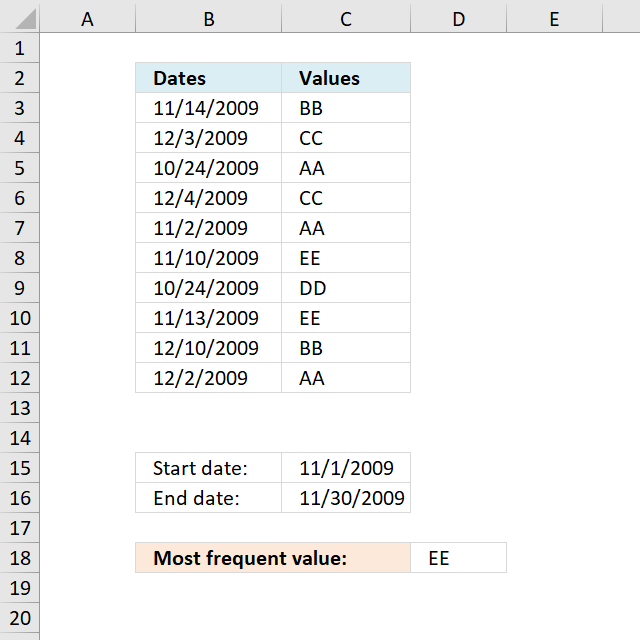Author: Oscar Cronquist Article last updated on December 07, 2018In this article I will show you how to extract the most frequent value (text or number) between two dates in a column.

Array formula in D18:

=INDEX(\$C\$3:\$C\$12, MATCH(MODE.SNGL(IF((\$B\$3:\$B\$12<=\$C\$16)*(\$B\$3:\$B\$12>=\$C\$15), COUNTIF(\$C\$3:\$C\$12, "<"&\$C\$3:\$C\$12), "")), COUNTIF(\$C\$3:\$C\$12, "<"&\$C\$3:\$C\$12),0))

To enter an array formula, type the formula in a cell then press and hold CTRL + SHIFT simultaneously, now press Enter once. Release all keys.

The formula bar now shows the formula with a beginning and ending curly bracket telling you that you entered the formula successfully. Don't enter the curly brackets yourself.

#### Step 1 - Calculate rank order if sorted

The COUNTIF function counts values based on a condition or criteria, the < less than sign makes the COUNTIF calculate a rank number if the list were sorted from A to Z.

COUNTIF(\$C\$3:\$C\$12,"<"&\$C\$3:\$C\$12)

becomes

COUNTIF({"BB"; "CC"; "AA"; "CC"; "AA"; "EE"; "DD"; "EE"; "BB"; "AA"},"<"&{"BB"; "CC"; "AA"; "CC"; "AA"; "EE"; "DD"; "EE"; "BB"; "AA"})

becomes

COUNTIF({"BB"; "CC"; "AA"; "CC"; "AA"; "EE"; "DD"; "EE"; "BB"; "AA"}, {"<BB";"<CC";"<AA";"<CC";"<AA";"<EE";"<DD";"<EE";"<BB";"<AA"})

and returns

{3;5;0;5;0;8;7;8;3;0}.

#### Step 2 - Check which values are in range

The IF function returns the rank number number based on a logical expression. It returns boolean value TRUE if the value is in the date range. If boolean value is FALSE the IF function returns "" (nothing).

IF((\$B\$3:\$B\$12<=\$C\$16)*(\$B\$3:\$B\$12>=\$C\$15),COUNTIF(\$C\$3:\$C\$12,"<"&\$C\$3:\$C\$12),"")

becomes

IF({1;0;0;0;1;1;0;1;0;0},COUNTIF(\$C\$3:\$C\$12,"<"&\$C\$3:\$C\$12),"")

becomes

IF({1;0;0;0;1;1;0;1;0;0}, {3; 5; 0; 5; 0; 8; 7; 8; 3; 0},"")

and returns

{3; ""; ""; ""; 0; 8; ""; 8; ""; ""}.

#### Step 3 - Calculate the most frequent number

The MODE.SNGL function returns the most frequent number in a cell range or array.

MODE.SNGL(IF((\$B\$3:\$B\$12<=\$C\$16)*(\$B\$3:\$B\$12>=\$C\$15),COUNTIF(\$C\$3:\$C\$12,"<"&\$C\$3:\$C\$12),""))

becomes

MODE.SNGL({3; ""; ""; ""; 0; 8; ""; 8; ""; ""})

and returns 8.

#### Step 4 - Find position of most frequent number in array

The MATCH function finds the relative position of a value in an array or cell range.

MATCH(MODE.SNGL(IF((\$B\$3:\$B\$12<=\$C\$16)*(\$B\$3:\$B\$12>=\$C\$15), COUNTIF(\$C\$3:\$C\$12, "<"&\$C\$3:\$C\$12), "")), COUNTIF(\$C\$3:\$C\$12, "<"&\$C\$3:\$C\$12),0)

becomes

MATCH(8, COUNTIF(\$C\$3:\$C\$12, "<"&\$C\$3:\$C\$12),0)

becomes

MATCH(8, {3; 5; 0; 5; 0; 8; 7; 8; 3; 0},0)

and returns 6.

#### Step 5 - Return value

The INDEX function returns a value based on row number (and column number if needed)

INDEX(\$C\$3:\$C\$12, MATCH(MODE.SNGL(IF((\$B\$3:\$B\$12<=\$C\$16)*(\$B\$3:\$B\$12>=\$C\$15), COUNTIF(\$C\$3:\$C\$12, "<"&\$C\$3:\$C\$12), "")), COUNTIF(\$C\$3:\$C\$12, "<"&\$C\$3:\$C\$12),0))

becomes

INDEX(\$C\$3:\$C\$12, 6)

and returns "EE" in cell D18.